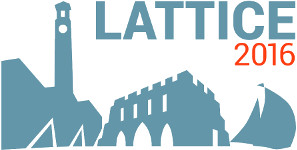#The 34th International Symposium on Lattice Field Theory (Lattice 2016)

Jul 24 – 30, 2016
Highfield Campus, University of Southampton
Europe/London timezone

## A method to compute derivatives of functions of large complex matrices

Jul 27, 2016, 12:10 PM
20m
Building 67 Room 1007 (Highfield Campus, University of Southampton)

### Building 67 Room 1007

#### Highfield Campus, University of Southampton

Talk Algorithms and Machines

### Speaker

Mr Matthias Puhr (University of Regensburg)

### Description

We present a method for the numerical calculation of derivatives of functions of general complex matrices which also works for implicit matrix function approximations such as Krylov-Ritz type algorithms. An important use case for the method is the overlap Dirac operator at finite quark chemical potential. The evaluation of the overlap Dirac operator at finite chemical potential calls for the computation of the product of the sign function of a non-Hermitian matrix with some source vector. For non-Hermitian matrices the sign function can no longer be efficiently approximated with polynomials or rational functions. Instead one invokes implicit approximation algorithms, like Krylov-Ritz methods, that depend on the source vector. Our method allows for an efficient calculation of the derivatives of such implicit approximations, which is necessary for the computation of conserved lattice currents or the fermionic force in Hybrid Monte-Carlo or Langevin simulations. We also give an explicit deflation prescription for the case when one knows several eigenvalues and eigenvectors of the matrix being the argument of the differentiated function. To show that the method is efficient and well suited for practical calculations we provide test results for the two-sided Lanczos approximation of the finite-density overlap Dirac operator on $SU(3)$ gauge field configurations on lattices with sizes up to $14\times14^3$

### Primary author

Mr Matthias Puhr (University of Regensburg)

### Co-author

Dr Pavel Buividovich (University of Regensburg)

 Slides